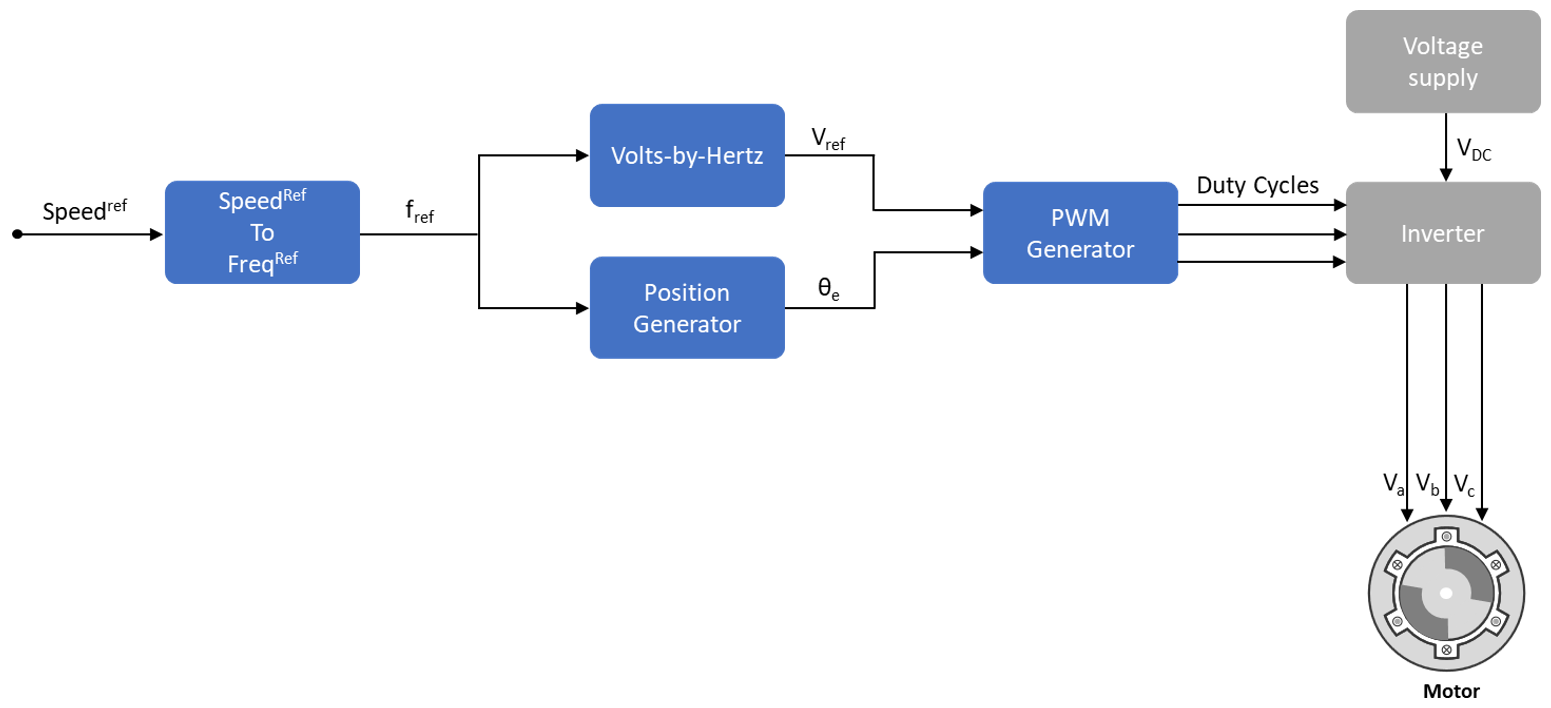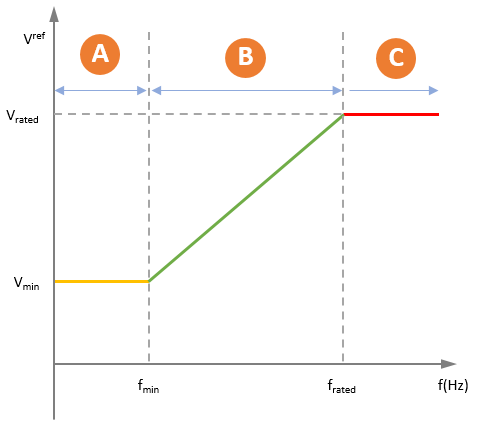## Open-Loop and Closed-Loop Control

This section describes the open-loop and closed loop motor control techniques.

### Open-Loop Motor Control

Open-loop control (also known as scalar control or Volts/Hz control) is a popular motor control technique that you can use to run any AC motor. This is a simple technique that does not need any feedback from the motor.This figure shows an open-loop control system. The power circuit consists of a PWM voltage fed inverter supplied by a DC source. The system does not use any feedback signal for control implementation. It uses the reference speed to determine the frequency of the stator voltages. The system computes the voltage magnitude as proportional to the ratio of rated voltage and rated frequency (commonly known as Volts/Hz ratio), so that the flux remains constant.

where:

• ${\lambda }_{m}$ is the rated flux of the motor in Wb.

• ${V}_{s}$ is the stator voltage of the AC motor in Volts.

• ${f}_{s}$ is the frequency of the stator voltage of the AC motor in Hz.

In an open-loop system, the speed for an AC motor is expressed as:

where:

• $Spee{d}_{\left(rpm\right)}$ is the mechanical speed of the AC motor in rpm.

• ${f}_{s}$ is the frequency of the stator voltage and currents of the AC motor in Hz.

• $p$ is the number of pole pairs of the motor.

Use this equation to determine the frequency of the reference voltages, fref, needed to reach a required reference speed, RPMref (for a given machine).

Use this frequency to generate PWM reference voltages for the inverter. Compute the magnitude of the voltages by maintaining the Volts/Hz ratio as:

The system keeps the ratio of rated voltage to rated frequency (Vrated / frated) constant when computing the peak amplitude of PWM reference voltages (Vref) from the reference voltage frequency (fref). By keeping the voltage / frequency ratio constant (or Vref proportional to fref) for three-phase voltage waveforms, the stator magnetic flux remains relatively constant. However, as shown in this figure, the V/F ratio does not remain constant throughout the entire range of motor speed.• Region A — At lower speeds, the system needs a minimum boost voltage (15% or 25% of the rated voltage) to overcome the effect of the stator resistance voltage drop. The voltage Vmin acts as a compensation for this drop.

• Region B — In this region, the system keeps the Vrated / frated ratio constant. It follows V/F control by computing Vref according to fref.

• Region C — At higher speeds, the system cannot maintain a constant V/F ratio because the stator voltages are limited to Vrated. In this region, Vref remains equal to Vrated even when there is a rise in fref.

When using the per-unit system representation, the open-loop control system considers Vrated as the base quantity, which usually corresponds to 1PU or 100% duty cycle. Depending on the modulation technique (either Sinusoidal PWM or Space Vector PWM), you may need an additional gain ($\left(\frac{2}{\sqrt{3}}\right)$ for sinusoidal PWM).

You can use open-loop control in applications where dynamic response is not a concern, and a cost-effective solution is required. Open-loop motor control does not have the ability to consider external conditions that can affect the motor speed. Therefore the control system cannot automatically correct the deviation between the desired and the actual motor speeds.

Note

Scalar control implementation does not consider compensating voltage drop due to stator resistance and field weakening.

### Closed-Loop Motor Control

Closed-loop control takes the system feedback into consideration for control. Closed-loop control of the motor considers the feedback of motor signals like current and position. The control system uses the feedback signals to regulate the voltage (applied to the motor) to keep the motor response at a reference value.Field-Oriented Control (FOC) (or vector control) is a popular closed-loop system that is used in motor control applications. The FOC technique is used to implement closed-loop torque, speed, and position control of motors. This technique also provides good control capability over the full torque and speed ranges. The FOC implementation needs transformation of stator currents from the stationary reference frame to the rotor flux reference frame.

Speed control and torque control are the commonly used control modes in FOC. The position control mode is less commonly used. Most traction applications use the torque control mode in which the motor control system follows a reference torque value. In the speed control mode, the motor controller follows a reference speed value and generates a torque reference for torque control that forms an inner subsystem. Whereas, in the position control mode, the speed controller forms the inner subsystem.

You need real-time feedback of the current and rotor position to implement the FOC algorithm. You can use sensors to measure the current and the rotor position. You can also use sensorless techniques that use estimated feedback values instead of the actual sensor-based measurements.

Closed-loop control uses the real-time position and stator current feedback to tune the speed controller and the current controller and change the duty cycles of the inverter. This ensures that the corrected three-phase voltage supply (that runs the motor) corrects the motor feedback deviation from the desired value.

### Open-Loop to Closed-Loop Transitions

Some applications require the motor to start using an open-loop control. Once the motor achieves the minimum required stability in open-loop control, the control system shifts to closed-loop.

In a quadrature encoder-based position sensing system, the motor starts up in open-loop and transitions to closed-loop once the index pulse is detected.

In sensorless position control, the motor starts running at 10% of the base speed in the open-loop. After the reference switch goes beyond 10% of the base speed, the control system transitions from open-loop to closed-loop.

To ensure smooth transition from open-loop to closed-loop, the PI controllers reset and start from the same initial condition as the open-loop outputs.